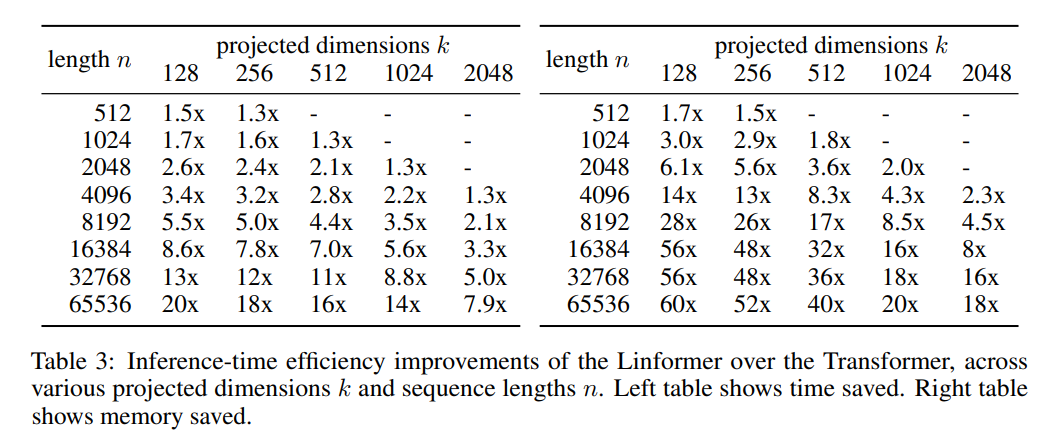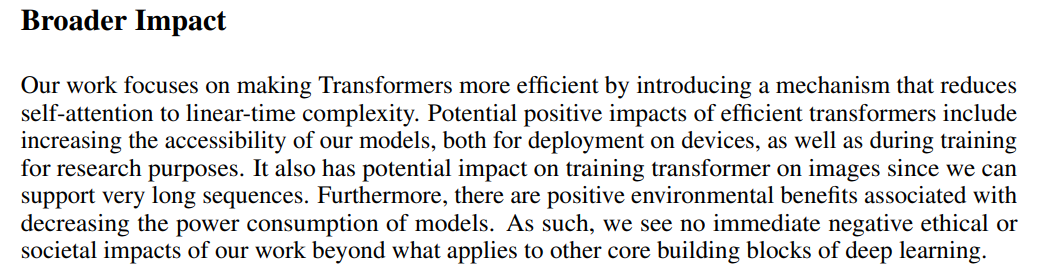## Paper Review: Linformer: Self-Attention with Linear Complexity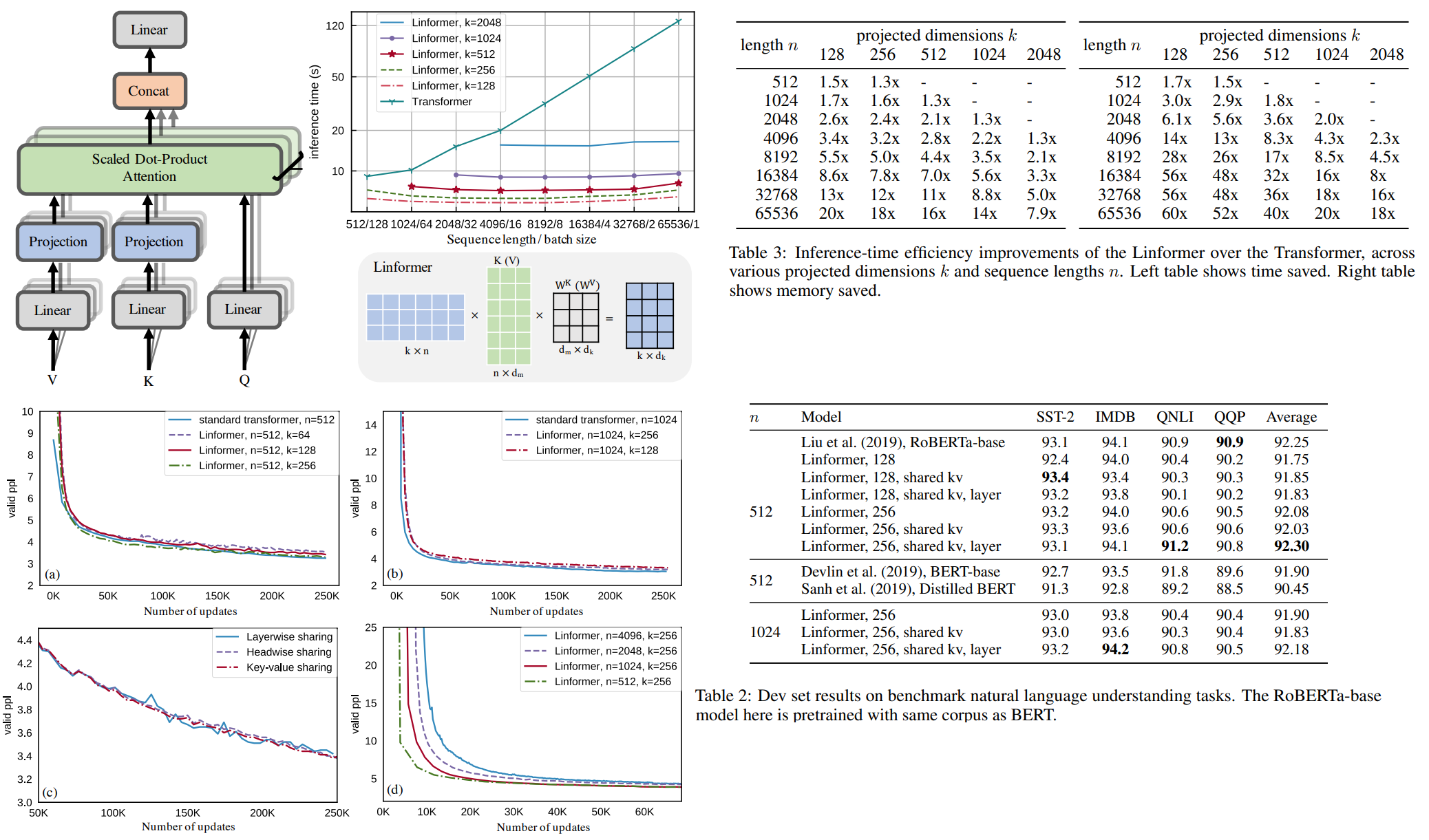The authors realized that self-attention can be approximated by a low-rank matrix. So they offer a new self-attention architecture, which reduces compexity from O(N^2) to O(N) in both time and space.

Very efficient… but still trains on 64 V100.

Authors decompose the original scaled dot-product attention into multiple smaller attentions through linear projections, such that the combination of these operations forms a low-rank factorization of the original attention.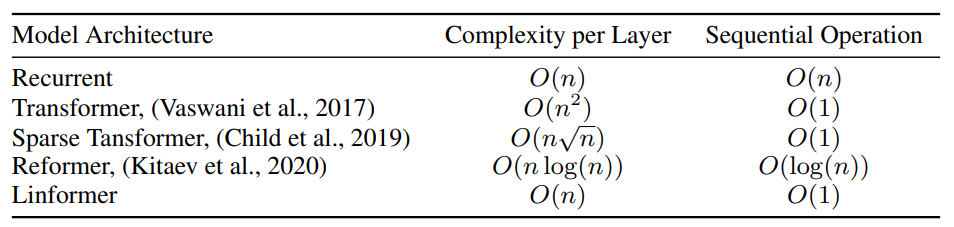### Self-Attention is Low Rank

P is the context mapping matrix.

Authors take RoBERTa-base and RoBERTa-large pretrained on classification and masked-language-modeling tasks. They apply SVD into P across heads and layers of the model and plot the normalized cumulative singular value averaged over 10k sentences. That means, that most information can be gained by taking first few largest singular values.

We could approximate P with low-rank matrix. But this would mean doing SVD in each self-attention matrix, so authors offer another idea.### Model

The main idea is adding two linear projection matrices (E, F) when computing key and value. They take KW and VW `n x d` and project into dimension `k x d`, then compute `n x k` matrix P using scaled attention.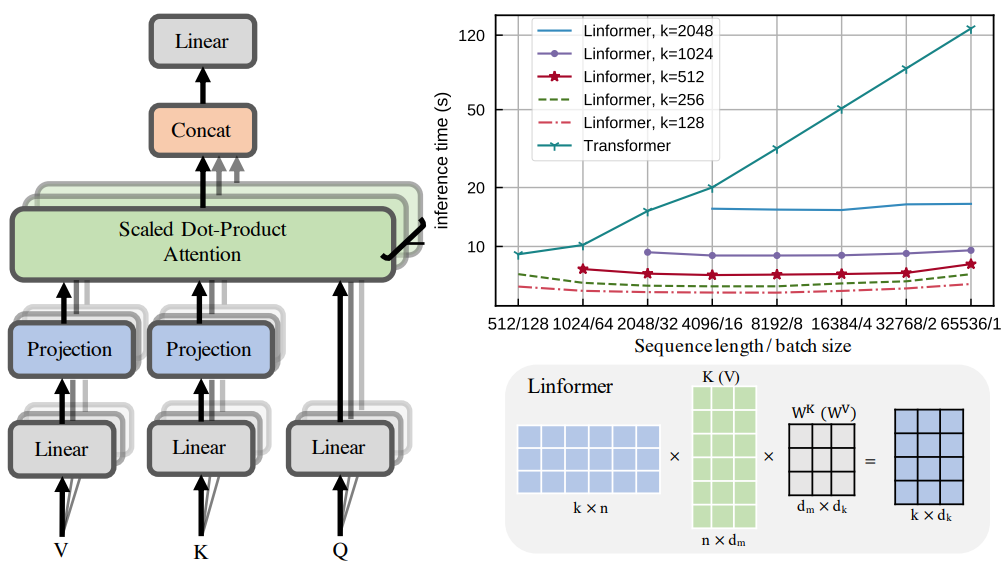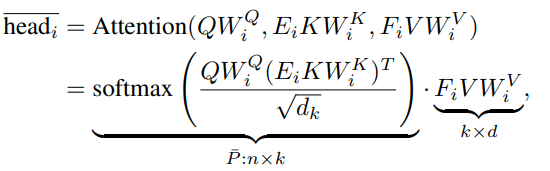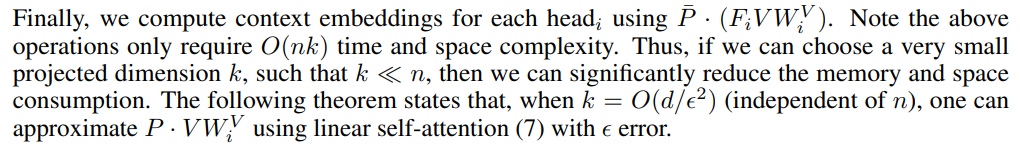• Parameter sharing between projections: Headwise, layerwise or key-value sharing
• Nonuniform projected dimension. It could be efficient to set lower projection dimension for higher levels
• General projections. Some different kind of projection instead of linear - pooling or convolution with kernel `n` and stride `k`

### Experiments

RoBERTa. 64 Tesla V100 GPUs with 250k updates.

First of all, we can see that validation perplexity is almost the same as in transformer.

Note that as sequence length increases, even though projected dimension is fixed, the final perplexities after convergence remain about the same.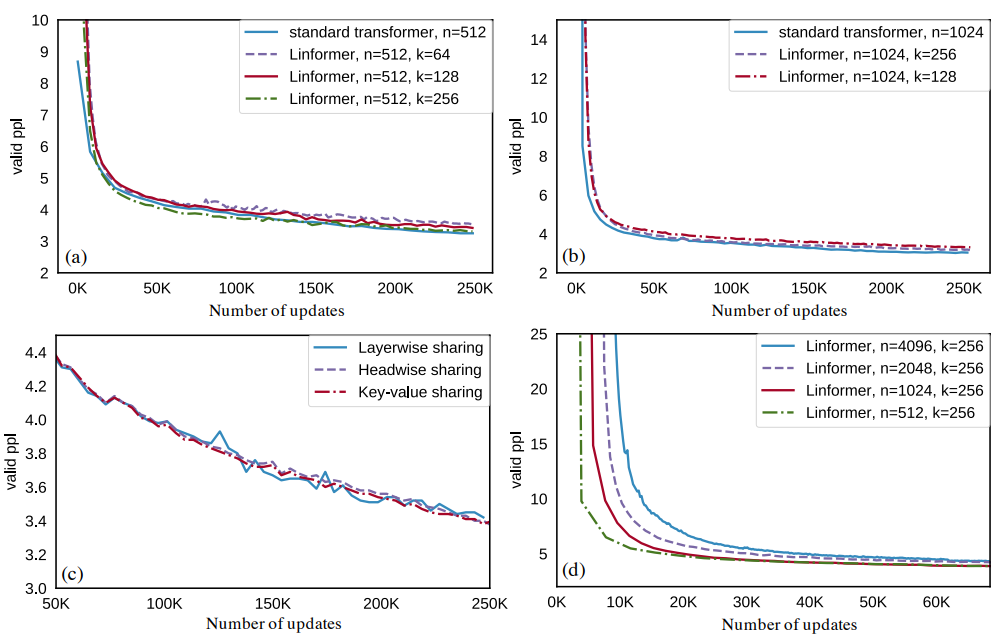In downstream tasks quality is almost the same. In some cases even better!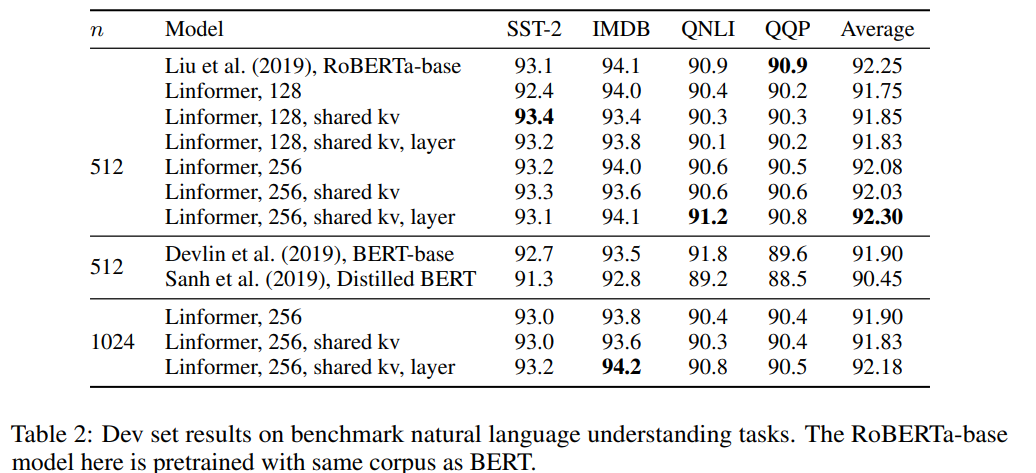And inference is much faster than transformer. And requires less memory.